# Ounces-to-Gallons Method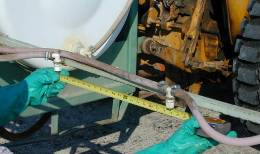This method uses a linear calibration course equal to 1/128th of an acre. The output from the sprayer is collected for the time required to travel 1/128th of an acre. Because a gallon is 128 ounces and the course is 1/128th of an acre, the output collected in ounces is equal to the gallons applied per acre. The Travel Distance Table shows how many linear feet the sprayer must travel to give the equivalent of 1/128th of an acre at various nozzle spacings and band or row widths. When the sprayer travels this distance, each nozzle will have sprayed 1/128th of an acre.

### Step 1: Distance

Using the Travel Distance Table, find the distance (in feet) you need to travel for your nozzle spacings, band or row widths (in inches). Use nozzle spacing (distance between nozzles on boom) to determine driving distance for broadcast sprays. Use band width (width of the wetted band beneath the nozzle) to determine travel distance for banded sprays (including post-directed herbicide sprays). Use row width for foliar sprays directed to the crop. Mark off this distance in a field, allowing space for the sprayer to reach full operating speed before reaching the first marker of the test course.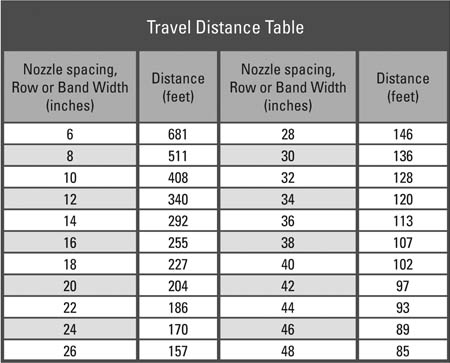To calculate travel distance for spacings or widths not listed, use the following formula: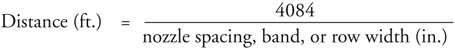### Step 2: Time

Fill the tank half full with water (no pesticide). Make at least two runs over the marked distance with all the equipment (cultivator, disk, planter, etc.) that will be used during the application engaged. Select RPM and gear settings, and record the average time in seconds that the sprayer takes to travel the marked distance without spraying.

### Step 3: Output Collection/GPA

Park the tractor and adjust sprayer pressure to the level that will be used for application. Using a container marked in ounces, catch the output from a single nozzle for the length of the average travel time (from Step 2). The number of ounces collected from one nozzle is equal to the output of the entire sprayer in gallons per acre (GPA). (Because you have already determined that output from each nozzle is within 10 percent of the average output as described in the Pre-calibration Checkup section, it is not necessary to collect output from every nozzle during this step).

Note: When more than one nozzle is used per row or band, the spray collected from one nozzle should be multiplied by the number of nozzles directed at the row or band. All nozzles in this group must be the same size and have uniform output. This combined output, in ounces, from all nozzles directed at the row or band is equal to the gallons per acre being applied to that row.

If necessary, make adjustments to obtain desired output. Change pressure for small adjustments. Change speed (and re-calibrate) or nozzle tips for large adjustments.

### Step 4. How Much to Use

After calibrating the equipment, figure the amount of pesticide and carrier to put in the tank to get the desired application rate based on the application method. See the examples for various application methods.

If you will be using a carrier other than water, the GPA number you get from following Steps 1, 2, and 3 can be adjusted by using a conversion factor from the table below. Multiply the GPA value obtained with water during the calibration with the conversion factor for the solution being sprayed to get the correct output.  For example, after calibrating your sprayer using water you determine the output to be 20 gallons per acre.  If you will be applying the pesticide with 28% nitrogen solution, the conversion factor is .89, and you will multiply 20 X .89 to find an actual application rate of 17.8 GPA.

 Weight of Solution in pounds per gallon Conversion Factor 6.6 (kerosene) 1.26 7.0 1.09 8.0 1.02 8.34 (water) 1.00 9.0 .96 10.0 .91 10.65 (28% nitrogen solution) .89 10.8 (30% nitrogen solution) .88 11.0 (7-27-7 fertilizer) .87 11.06 (32% nitrogen solution) .87 11.4 (10-34-0 fertilizer) .86 11.5 (12-0-26) .85 11.6 (11-37-0 fertilizer) .85 12.0 .83 14.0 .77

#### Example 1

Broadcast herbicide application on a 30 acre field at a rate of 2 pints per acre. Nozzle spacing is 22 inches.

Step 1. — Based on a 22-inch nozzle spacing, you need to drive 186 feet.

Step 2. — You determine that it takes 32 seconds to drive 186 feet.

Step 3. — Output per nozzle for 32 seconds is 13 ounces. This means your sprayer is applying 13 gallons per acre.

Step 4. — To figure out how much herbicide is needed to treat the field:

Multiply the number of acres (30) by the gallons-per-acre output (13) to determine the amount of total spray solution needed to treat the field:

30 acres × 13 gallons per acre = 390 gallons spray solution.

The amount of herbicide needed to treat the field is:

30 acres × 2 pints per acre = 60 pints or 7.5 gallons.

Add 7.5 gallons of herbicide to 382.5 gallons of water (390-7.5) to treat the 30-acre field.

#### Example 2

Banded herbicide application in 12-inch band over corn rows spaced 36 inches apart. Field size is 30 acres and labeled (broadcast) rate is 2 pints per acre. The band is applied with one nozzle per row.

Step 1. — Based on a band width of 12 inches, you need to drive 340 feet.

Step 2. — You determine that it takes 58 seconds to drive 340 feet.

Step 3. — Output per nozzle for 58 seconds is 24 ounces. This means your sprayer is applying 24 gallons per treated acre.

Note: When you apply pesticides in a band, you are treating only a fraction of the total area of the field. This area is referred to as treated acres. To determine how many treated acres there are in a specified field acreage, multiply the field acreage by the ratio of the band width to the row width: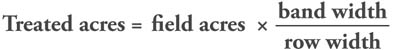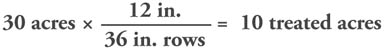Step 4. — To figure out how much herbicide is needed to treat the field:

Multiply the number of treated acres by the gallons per treated acre to determine the amount of total spray solution needed to treat the entire field:

10 treated acres × 24 gallons per treated acre = 240 gallons spray solution.

Determine the amount of herbicide needed to treat the 30-acre field by multiplying the number of treated acres by the labeled broadcast rate:

10 treated acres × 2 pints per acre = 20 pints or 2.5 gallons

Add 2.5 gallons of herbicide to 237.5 gallons of water (240-2.5) to treat the 30-acre field in 12-inch bands.

#### Example 3

Directed fungicide application using 3 nozzles per row to a crop planted in 40-inch rows. The field size is 30 acres and the labeled (broadcast) rate is 2.5 quarts per acre.

Step 1. — Based on a row spacing of 40 inches, you need to drive 102 feet.

Step 2. — You determine that it takes 17 seconds to drive 102 feet.

Step 3. — Output per group of 3 nozzles directed to the row for 17 seconds is 18 ounces (6 ounces per nozzle). This means your sprayer is applying 18 gallons per acre.

Step 4. — To calculate how much fungicide is needed to treat the field:

Multiply field acres by the gallons-per-acre output to determine the amount of total spray solution needed to treat the field:

30 acres × 18 gallons per acre = 540 gallons spray solution.

The amount of fungicide needed to treat the field is:

30 acres × 2.5 quarts per acre = 75 quarts or 18.75 gallons.

Add 18.75 gallons of fungicide to 521.25 gallons of water (540-18.75) to treat the 30-acre field.

Compiled by Wayne Buhler, PhD.